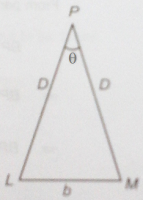# Units, Measurements, Error Analysis and Dimensions - Notes on Measurements

In continuation of section wise notes from the chapter Units, Measurements, Dimensions and Error Analysis containing important study materials and more it goes ...

Improve your Performance & Confidence through -

### Measurement

What is Measurement?
Measurement is the comparison of a quantity with a standard of the same physical quantity. (How do we define Physical Quantity?) In other words, measurement of a physical quantity requires is comparison with an arbitrarily chosen, reference standard which is called unit of that quantity.

Measurement of Large Distances -
Large distances, for example distance between stars, planet are measured by indirect methods. Hence, an indirect method of measurement or making rough estimates of quantities using common observations is equally important.
Article continues after the Ad -

Parallax MethodParallax can be defined as the apparent shift in the positron of a body with respect to a specific point on its background with the shift of eye. Let us understand this in this way -
Suppose we want to measure the distance of a very distant planet (P) using Parallax Method, we observe it from two different observatories (L & M) on the earth separated by a distance say, 'b' which is called "basis". Since the planet is too far, both the observatories L & M can be considered at nearly the distance from it.
LP = MP = D (say). Also, 'b' is too less as compared to 'D'.Thus LM can be approximately considered as an arc of length 'b' of a circle whose center less at P. The radius of the circle is then, D. So, the angle subtended by LM at P can be used to find D using the relation:
q = b / D
Angle q is called the Parallax angle or parallactic angle and it should be taken in radian, not in degree.
After calculating the distance of the planet from the earth using Parallax Method, we can also calculate the size of the planet by using the following relation -
d = Df
Where, the angle f is the angular size or angular diameter of the planet.

Measurement of Very Small Distances -
Besides distance / length of normal magnitudes, we can do direct measurements of very small lengths (minute size) of the scale of molecular size using specific instruments like:
Optical Microscope - works in visible light & can resolve particles up to 10-7 m.
Electron Microscope - uses electron beams focussed by electric and magnetic field, better resolution power than that of optical microscope, can measure as small as 0.6 x 10–10 m.
Tunnelling Microscope - has better resolution power than electron microscope, can measure the size of a molecule.
Volumetric Method - this method is used to estimate the sizes of large molecules e.g., oleic acid.

Measurement of Mass
 Other Topics from this Chapter Dimensions, Dimensional Formulae, Dimensional Equation Dimensional Analysis and its Applications
Mass is a measure of the quantity of matter present in a body. It is one of the basic properties of matter. Variations in conditions such as pressure, temperature, location, etc. do not change the mass of an object. We need to employ different methods to measure masses of different ranges. For example -
Mass of Small Objects - We can use common balance, spring balance, pedestal balance etc. to find masses of common objects whereas, to estimate very small masses like molecular mass, we have to use analytical tools like Mass Spectrograph
Mass of Terrestrial Objects - calculated mathematically using Newton’s Law of Gravitation

Table: Range or Order of Masses
 Object Range of Mass (kg) Electron 10–30 Proton 10–27 Uranium atom 10–25 Red Blood Cell 10–13 A Dust Particle 10–9 Rain Drop 10–6 Human Body 102 Boeing 747 Aircraft 108 Moon 1023 Earth 1025 Sun 1030 Milky Way Galaxy 1041

Measurement of Time -
Any periodically recurring event can be used as a clock or as a standard of time. The periodic vibrations produced in a cesium atom serve as the most accurate standard of time. A cesium clock is also known as an Atomic Clock is based on these periodic vibrations. Atomic clocks have a high accuracy of ‘1 part in 1013’ i.e., they lose or gain no more than 3 ms (3x10–6 s) in a year. The national standard time interval ‘second’ and its frequency is maintained with a set of four cesium atomic clocks.

Units, Dimensions, Measurements and Error Analysis - Solved Test Series, Practice Questions